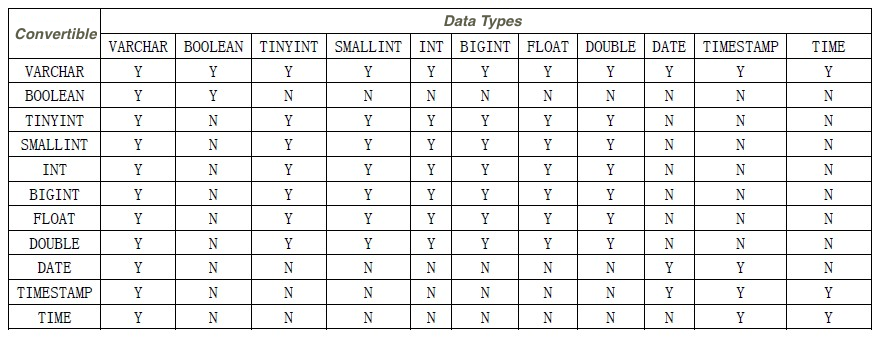This topic describes the data types that are supported by Realtime Compute for Apache Flink and how to convert between different data types.

## Data types supported by Realtime Compute for Apache Flink

Data type Description Value range
VARCHAR Stores strings of varying length. A VARCHAR string can store a maximum of 4 MB of data.
BOOLEAN Stores logical values. Valid values: TRUE, FALSE, and UNKNOWN.
TINYINT Stores tiny integers. Each tiny integer occupies 1 byte. `-128 to 127`.
SMALLINT Stores small integers. Each small integer occupies 2 bytes. `-32768 to 32767`.
INT Stores integers. Each integer occupies 4 bytes. `-2147483648 to 2147483647`.
BIGINT Stores big integers. Each big integer occupies 8 bytes. `-9223372036854775808 to 9223372036854775807`.
FLOAT Stores single-precision floating-point numbers. Each single-precision floating-point number occupies 4 bytes. Each single-precision floating-point number is accurate to six decimal places.
DECIMAL Stores the numbers that have the fixed precision and scale. The precision specifies the total number of digits both to the left and to the right of the decimal point. The scale specifies the number of digits to the right of the decimal point. For example, the value of `DECIMAL(5,2)` is `123.45`.
DOUBLE Stores double-precision floating-point numbers. Each double-precision floating-point number occupies 8 bytes. Each double-precision floating-point number is accurate to 15 decimal places.
DATE Stores dates. Example value: `DATE'1969-07-20'`.
TIME Stores time. Example value: `TIME '20:17:40'`.
TIMESTAMP Stores timestamps. Each timestamp contains both date and time. Example value: `TIMESTAMP '1969-07-20 20:17:40'`.
VARBINARY Stores binary data. This type corresponds to the `byte[]` array.
Note The storage size for the VARBINARY data type is not limited.

## Data type conversion## Example

• Test data
var1 (VARCHAR) big1 (BIGINT)
1000 323
• Test statements
``````cast (var1 as bigint) as AA;
cast (big1 as varchar) as BB;``````
• Test results
AA (BIGINT) BB (VARCHAR)
1000 323